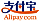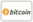Bookcover of Exact Solutions for a System of KdV Equations with Some Applications of White-Noise Analysis
Booktitle:

# Exact Solutions for a System of KdV Equations with Some Applications of White-Noise Analysis

LAP LAMBERT Academic Publishing (2016-07-01 )eligible for voucher
ISBN-13:

### 978-3-659-91328-0

ISBN-10:
3659913286
EAN:
9783659913280
Book language:
English
Blurb/Shorttext:
Applied functional analysis has many applications in other branches of mathematics, such as differential equations, numerical analysis, stochastic calculus, calculus of variations, quantum field theory, etc. Among of these applications, we interest in stochastic differential equations (SDEs). In particular, if we allow for some randomness in some of the coefficients of a partial differential equation (PDE), we often obtain a more realistic mathematical model of the situation. This model would be PDE involving stochastic parameters - stochastic partial differential equation (SPDE). Representative examples are the stochastic Korteweg-de Vries (KdV) equations.
Publishing house:
Website:
https://www.lap-publishing.com/
By (author) :
Hossam A. Ghany
Number of pages:
164
Published on:
2016-07-01
Stock:
Available
Category:
Mathematics
Price:
64.90 €
Keywords:
F-expansion method, Wick-type stochastic nonlinear differential equations, White noise theory, Solitary Wave Solution, Hermite transform### Categories